# How To Get Absolute Value in Google Sheets

Absolute value is the distance between a number and zero. Since distance can’t be negative, an absolute value is always a positive number, so as an example, the absolute value of 5 is 5 and the absolute value of -5 is also 5.Finding absolute values in Google Sheets can be useful for a variety of applications, but how do you do so without having to do it manually?

Fortunately, there are three simple ways to accomplish this task. In this article, I will walk you through three methods you can use to get absolute value in Google Sheets.

Contents

## How to Find Absolute Value in Google Sheets

Finding absolute values in Sheets is as easy as using one of three methods: the ABS function, the SUMPRODUCT function, or converting negative numbers to positives.

Take a look at how to use these three methods below.

### Using the ABS Function in Google Sheets

ABS is a function in Google Sheets that returns the absolute value of a number.

You can always just manually convert negative numbers to positive ones, and that would work fine if you were just trying to get an absolute value for one or two cells. However, imagine having a large spreadsheet with a table column that included 350 negative numbers.

Luckily, Google Sheets includes an ABS function so that you can quickly get absolute values for negative numbers without editing their cells. It’s a basic function that you can enter with this syntax: `=ABS(value)`. The ABS value can either be a cell reference or a number. For an example, follow these steps:

1. Open a blank spreadsheet in Google Sheets.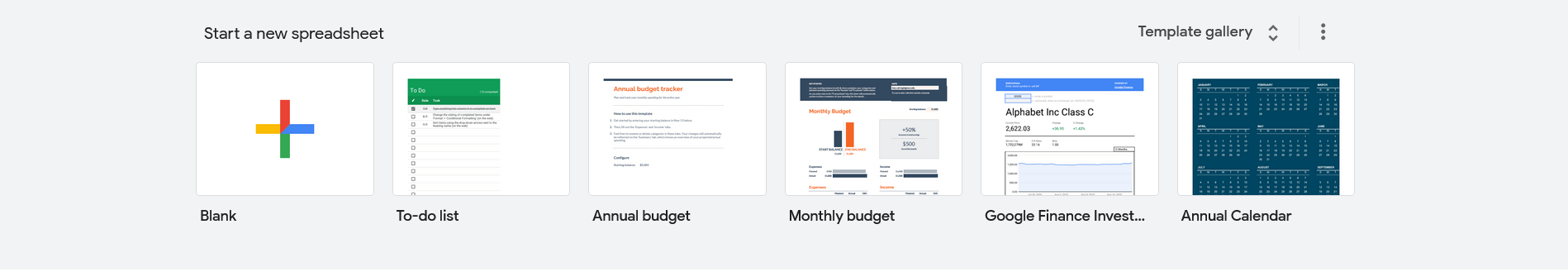2. Then enter the values ‘-454,’ ‘-250,’ and -‘350’ in the cells A2:A4 as shown in the snapshot directly below. Now you can convert that dummy data to absolute values.3. Select cell B2 and enter the function `=ABS(A2)` in the fx bar, and B2 will return the absolute value 454 when you press Enter.4. Copy the function into the other cells with the fill handle. Select B2, left-click the cell’s bottom right corner and drag the cursor over B3 and B4. Then, release the left mouse button to copy the ABS function into those cells as shown in the screenshot below.

ABS also calculates absolute values for the results of calculations. For example, select B5, enter `=ABS(A2+A4)` in the function bar, and press Return. B5 will return the absolute value of 804. The SUM function would return -804, but as an absolute value, the result is 804.

### Using the SUMPRODUCT Function in Google Sheets

ABS doesn’t add a range of numbers within a single cell reference. Furthermore, a cell range might include a mixture of positive and negative numbers. As such, a SUMPRODUCT combined with the ABS formula is perhaps the best way to add a series of numbers together to get an absolute value.

Before you add the SUMPRODUCT formula to your spreadsheet, enter ‘200’ in cell A5 and ‘300’ in A6. Then, enter the formula `=SUMPRODUCT(ABS(A2:A6))` in cell B6 and press Return. B6 now adds the cell range A2:A6 and returns an absolute value of 1,554.

You can also expand the formula so that it adds two or more cell ranges. Select cell B7 in your Sheets spreadsheet, and input the function `=SUMPRODUCT(ABS(A2:A6))+SUMPRODUCT(ABS(B2:B4))` in the function bar. The formula will add the numbers in the ranges A2:A6 and B2:B4 then return an absolute value total which in this case is 2,608.

### Convert Negative Numbers to Positive Numbers

Power Tools is a Sheets add-on with plenty of tools, including an option that converts number signs. To use this add-on, add Power Tools to Google Sheets, then follow these instructions to convert negative numbers to positive numbers:3. Select Power Tools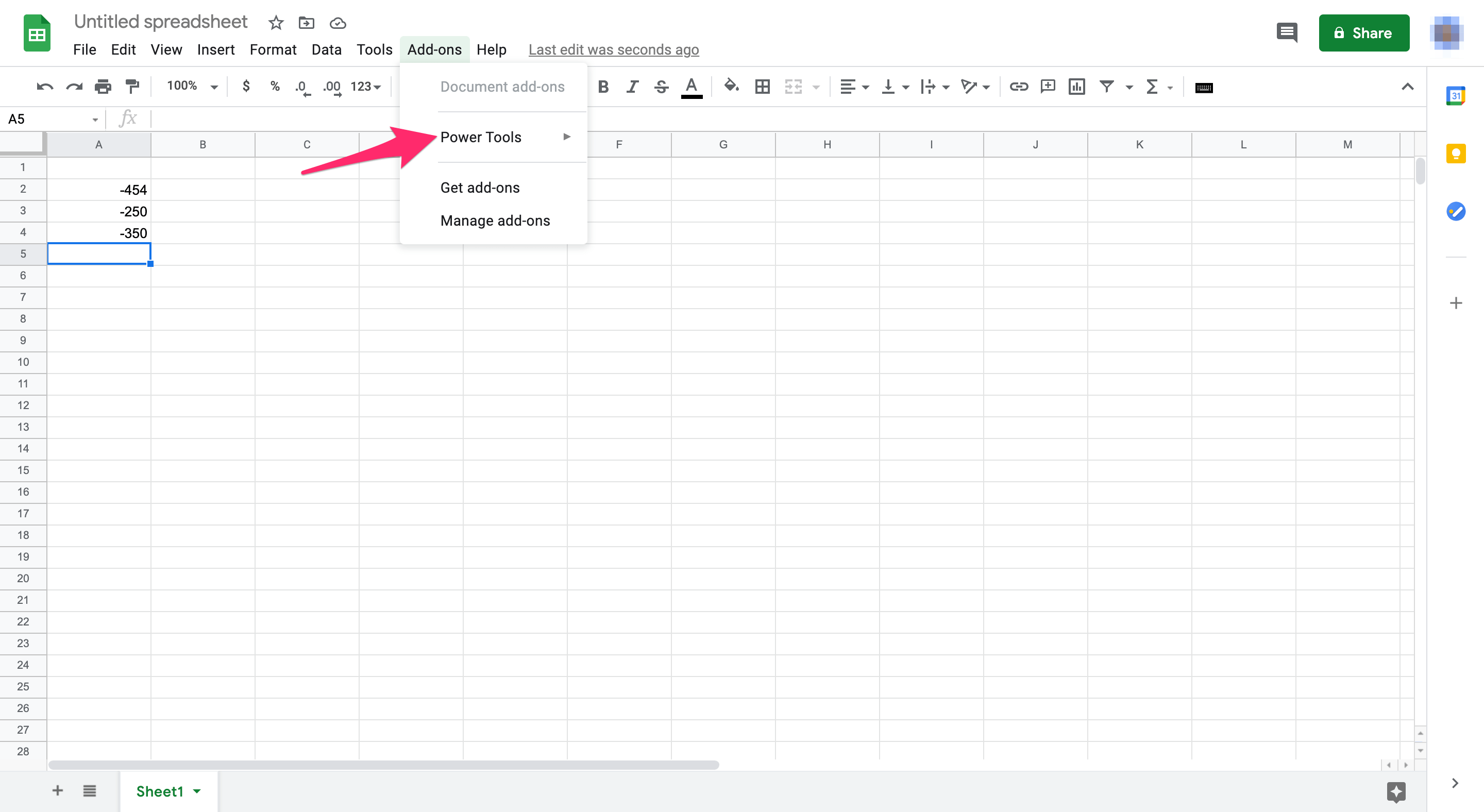4. Select Start from the pull-down menu to open Power Tools as in the screenshot below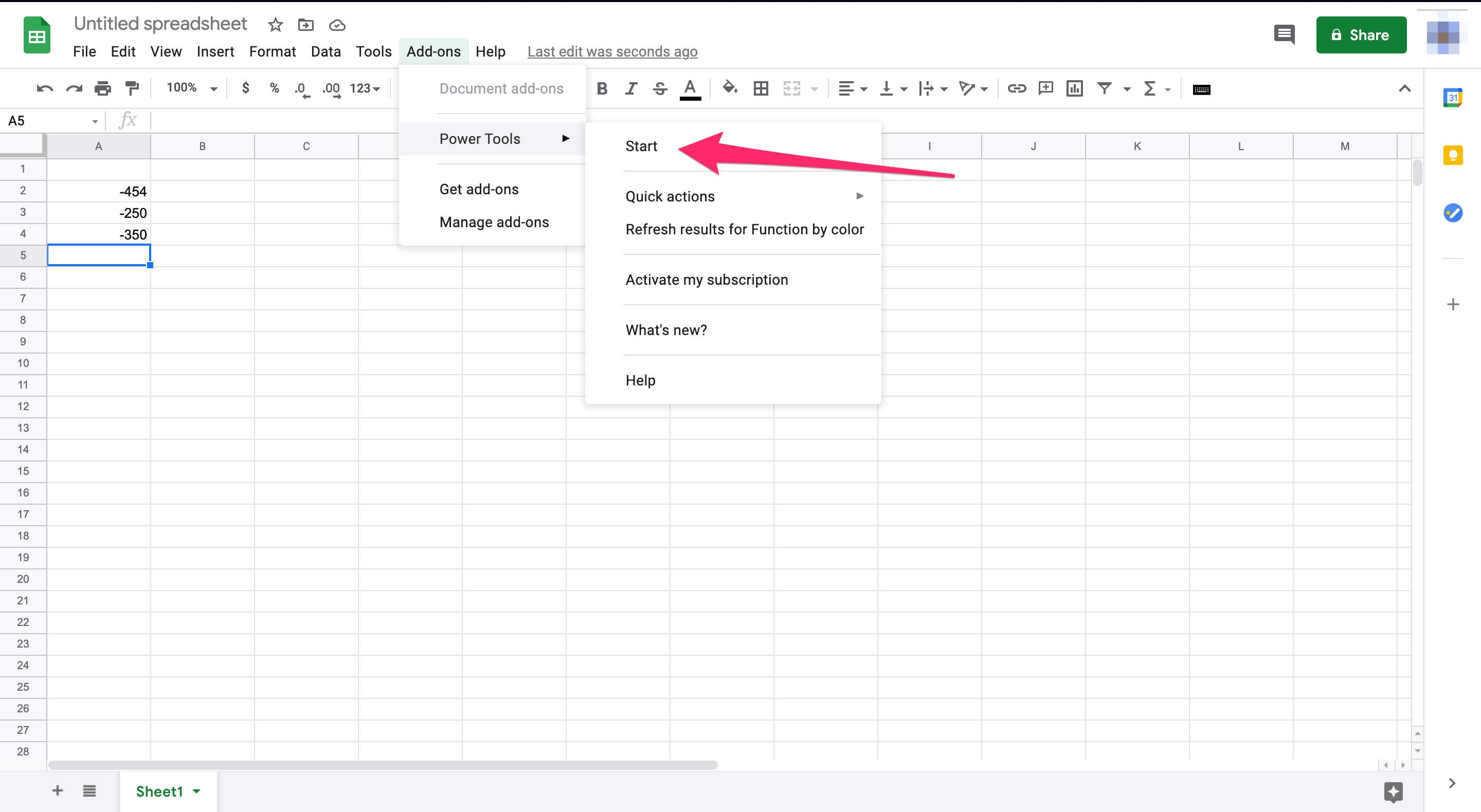5. Click Convert from the menu that opened on the right-hand side6. Click the Convert number sign checkbox.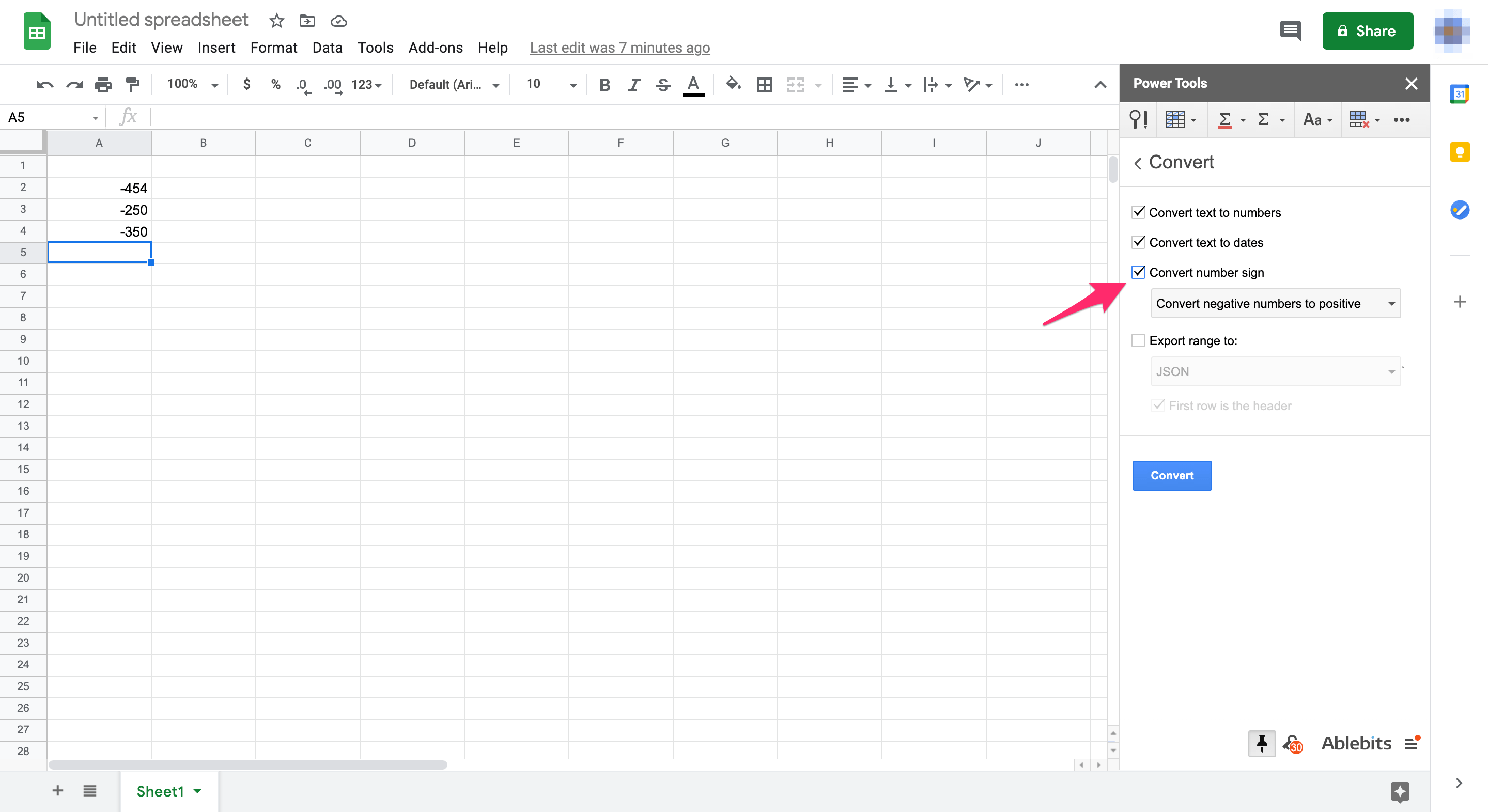7. Select Convert negative numbers to positive from the drop-down menu.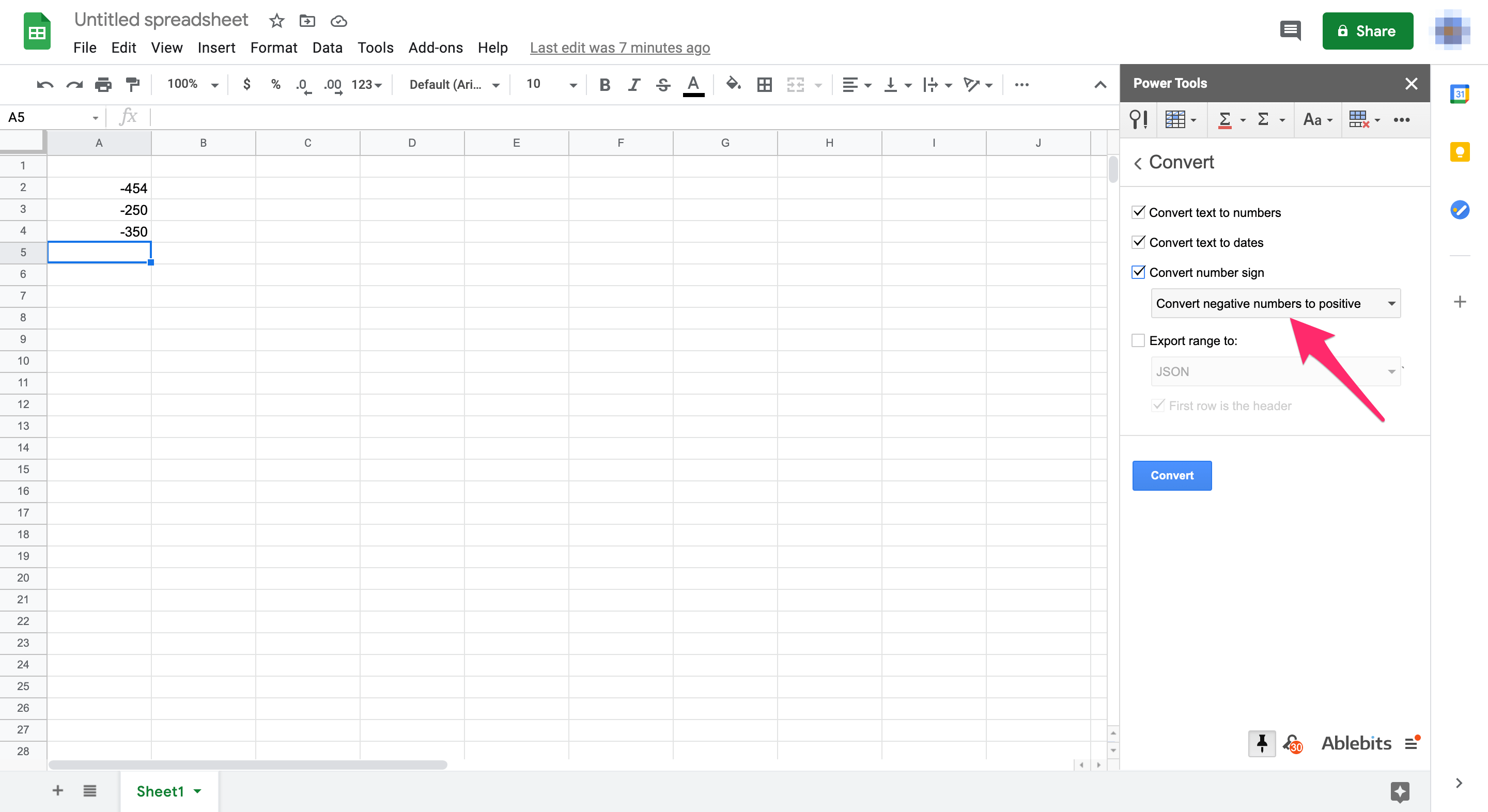8. Select the cell range A2:A4 on your Sheets spreadsheet with the cursor.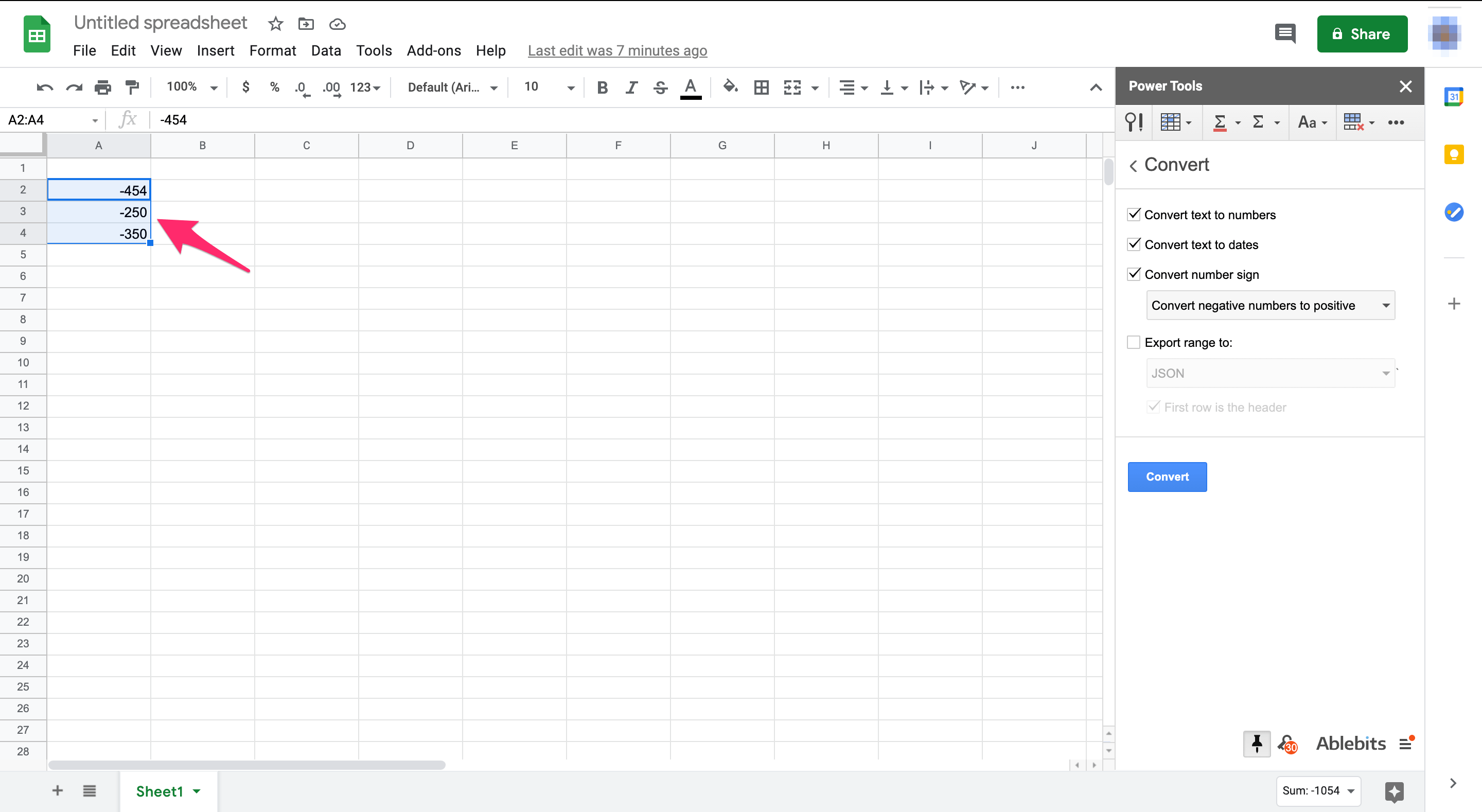9. Click the Convert button in the add-ons sidebarThis process removes the negative signs from the cells A2:A4 as shown in the screenshot below. Those cells now include absolute values rather than negative values. With this conversion option, you can quickly get the absolute values for a large range of cells without entering any ABS function in an adjacent column. The Power Tools Add-on has become an essential tool for Google Sheets power users.

## Final Thoughts

By following any of the above methods, you can get absolute values in Sheets without manually editing cells. If you use Excel, you might find How to get Absolute Value in Excel to be a useful  tutorial.

Do you have any Google Sheets tips and tricks that you find useful? Please comment below.

Sharing is caring!